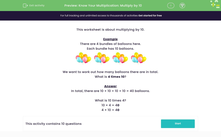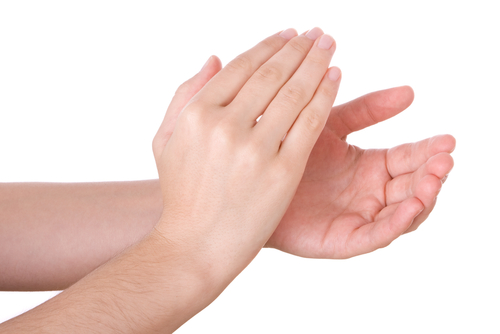# Know Your Multiplication: Multiply by 10

In this worksheet, students will multiply by 10.Key stage:  KS 1

Curriculum topic:   Number: Multiplication and Division

Curriculum subtopic:   Use Multiplication/Division Facts (2, 5, 10)

Popular topics:   Multiplication worksheets

Difficulty level:#### Worksheet Overview

This  activity is about multiplying by 10.

Example

There are 4 bundles of balloons here.

Each bundle has 10 balloons.We want to work out how many balloons there are in total.

What are 4 lots of 10?

In total, there are 10 + 10 + 10 + 10 = 40 balloons.

What is 4 x 10?

4 x 10 = 40

10 x 4 = 40

Let's try another example.

Below, there are 6 pairs of hands.

There are 10 fingers on each pair of hands.We want to know how many fingers there are in total.

What are 6 lots of 10?

In total, there are 10 + 10 + 10 + 10 + 10 + 10 = 60 pairs of hands.

What is 6 times 10?

6 x 10 = 60

10 x 6 = 60

Let's try some questions now.

### What is EdPlace?

We're your National Curriculum aligned online education content provider helping each child succeed in English, maths and science from year 1 to GCSE. With an EdPlace account you’ll be able to track and measure progress, helping each child achieve their best. We build confidence and attainment by personalising each child’s learning at a level that suits them.

Get started#### Popular Maths topics

••••